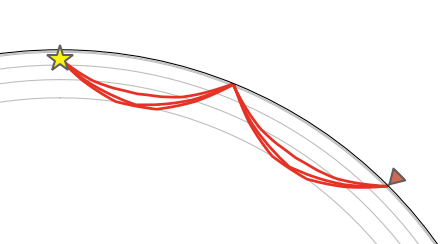# TauPy: getting unique times

I’m a novice Python user (ex Fortran and Matlab), so excuse me if this has an obvious answer.

I’d like to extract the travel times of P, pP, and PP phases, so I do this:

model=TauPyModel(model=‘iasp91’)
arrivals=model.get_travel_times(source_depth_in_km=121,distance_in_degree=45,phase_list=[“P”,“pP”,“PP”])
print(arrivals)
5 arrivals
P phase arrival at 483.090 seconds
pP phase arrival at 510.810 seconds
PP phase arrival at 590.651 seconds
PP phase arrival at 592.232 seconds
PP phase arrival at 595.152 seconds

But there are three times for PP.
Which is the “correct” one and how do I extract it?

They are all “correct” in that they consist of two mantle P phases - there is the direct one which I guess is the one you are expecting. The others are reflections and refractions on the 660 which is fairly strong in the IASP91 model:Please have a look at the phase naming documentation as well as the original paper:

Off the top of my head I’m not sure if its possible to exclude the other two PP phases.

1 Like